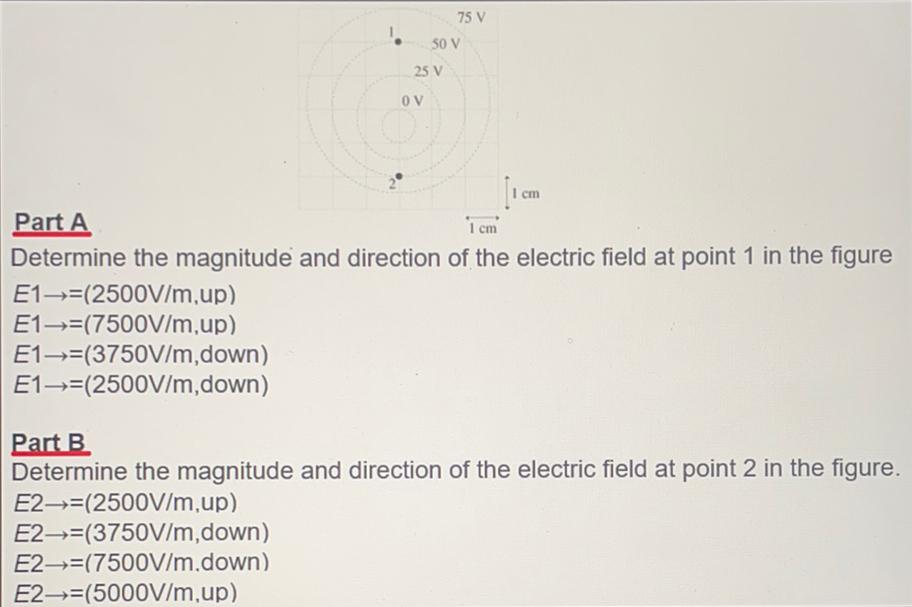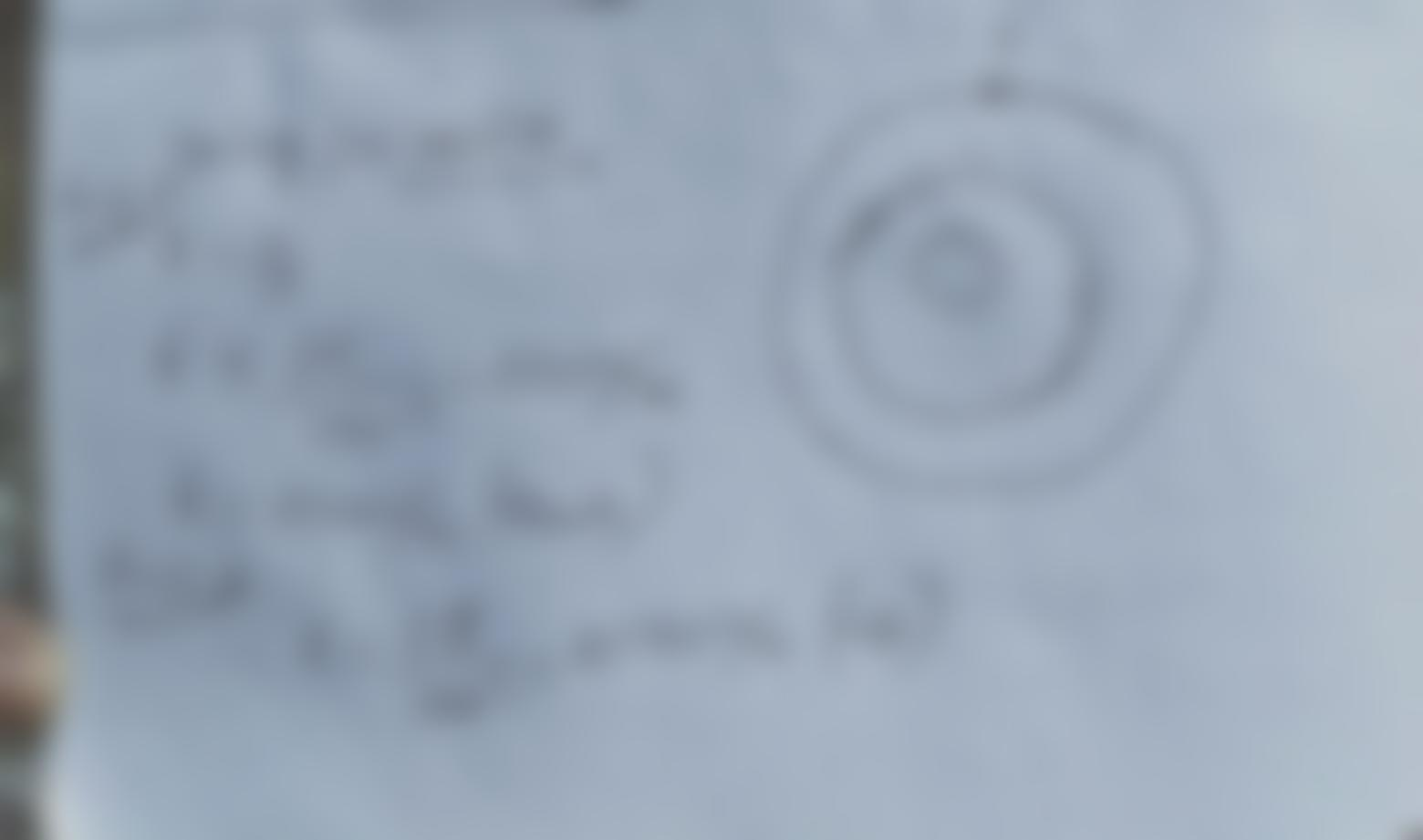Question:

# 75 V 50 V 25 V OV cm 1 cm Part A Determine the magnitude and direction of the electric field at point 1 in the figure E1=(2500V/75 V 50 V 25 V OV cm 1 cm Part A Determine the magnitude and direction of the electric field at point 1 in the figure E1=(2500V/m,up) E1+=(7500V/m,up) E1-→=(3750V/m, down) E1—-=(2500V/m, down) Part B Determine the magnitude and direction of the electric field at point 2 in the figure. E2-=(2500V/m,up) E2—-=(3750V/m, down) E2--=(7500V/m.down) E2-=(5000V/m,up)